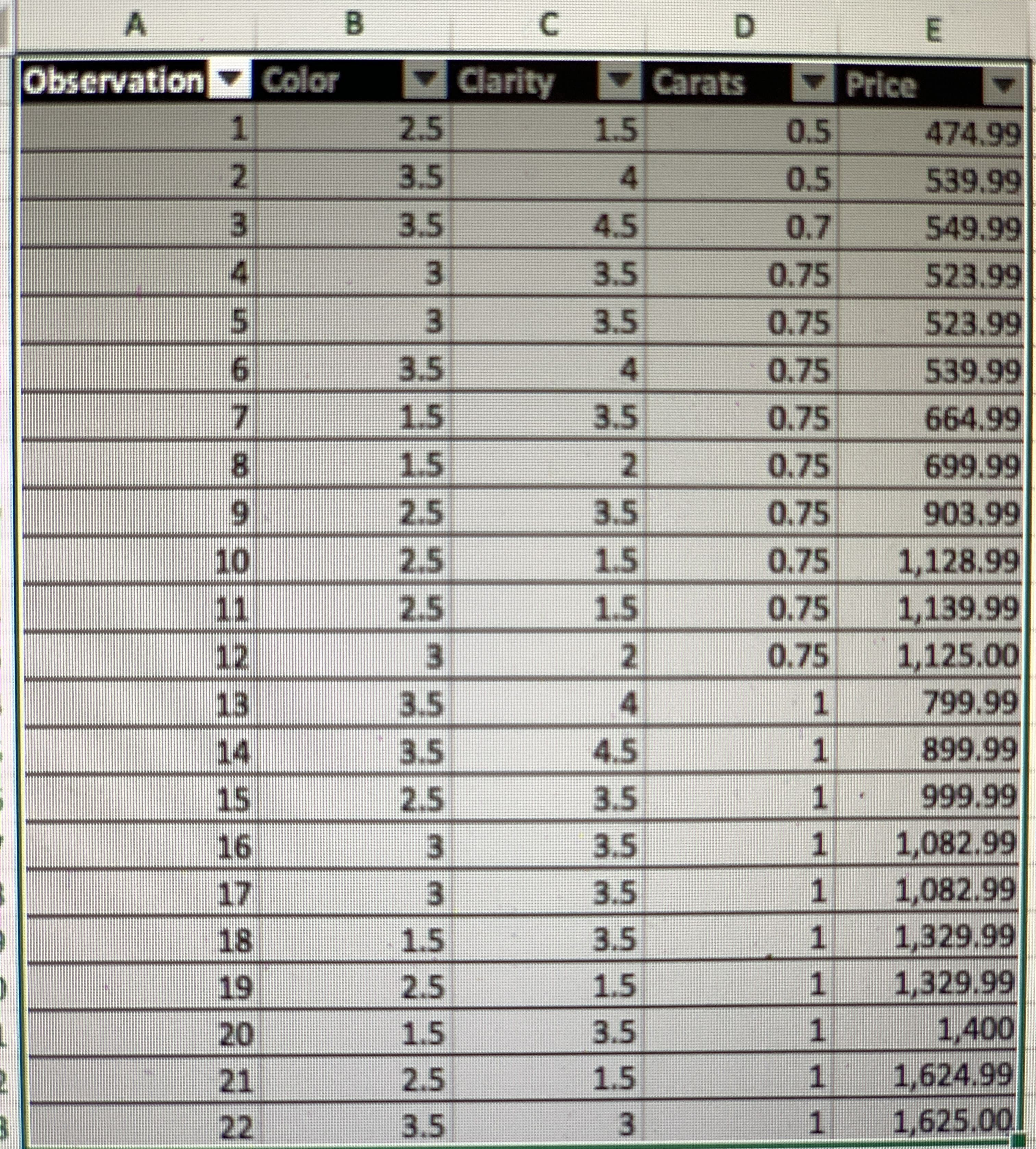Question# Case: Dr. Jung’s Diamonds Selection With Christmas coming, Dr. Jung became interested in buying diamonds for his wife. After perusing the Web, he lear

Scatterplots
ANSWEREDCase: Dr. Jung’s Diamonds Selection
With Christmas coming, Dr. Jung became interested in buying diamonds for his wife. After perusing the Web, he learned about the “4Cs” of diamonds: cut, color, clarity, and carat. He knew his wife wanted round-cut earrings mounted in white gold settings, so he immediately narrowed his focus to evaluating color, clarity, and carat for that style earring.
After a bit of searching, Dr. Jung located a number of earring sets that he would consider purchasing. But he knew the pricing of diamonds varied considerably. To assist in his decision making, Dr. Jung decided to use regression analysis to develop a model to predict the retail price of different sets of round-cut earrings based on their color, clarity, and carat scores. He assembled the data in the file Diamonds.xls for this purpose. Use this data to answer the following questions for Dr. Jung.
1) Prepare scatter plots showing the relationship between the earring prices (Y) and each of the potential independent variables. What sort of relationship does each plot suggest?
2) Let X1, X2, and X3 represent diamond color, clarity, and carats, respectively. If Dr. Jung wanted to build a linear regression model to estimate earring prices using these variables, which variables would you recommend that he use? Why?
3) Suppose Dr. Jung decides to use clarity (X2) and carats (X3) as independent variables in a regression model to predict earring prices. What is the estimated regression equation? What is the value of the R2 and adjusted-R2 statistics?
4) Use the regression equation identified in the previous question to create estimated prices for each of the earring sets in Dr. Jung’s sample. Which sets of earrings appear to be overpriced and which appear to be bargains? Based on this analysis, which set of earrings would you suggest that Dr. Jung purchase?
5) Dr. Jung now remembers that it sometimes helps to perform a square root transformation on the dependent variable in a regression problem. Modify your spreadsheet to include a new dependent variable that is the square root on the earring prices (use Excel’s SQRT( ) function). If Dr. Jung wanted to build a linear regression model to estimate the square root of earring prices using the same independent variables as before, which variables would you recommend that he use? Why?
1
6) Suppose Dr. Jung decides to use clarity (X2) and carats (X3) as independent variables in a regression model to predict the square root of the earring prices. What is the estimated regression equation? What is the value of the R2 and adjusted-R2 statistics?
7) Use the regression equation identified in the previous question to create estimated prices for each of the earring sets in Dr. Jung’s sample. (Remember, your model estimates the square root of the earring prices. So you must actually square the model’s estimates to convert them to price estimates.) Which sets of earring appears to be overpriced and which appear to be bargains? Based on this analysis, which set of earrings would you suggest that Dr. Jung purchase?
8) Dr. Jung now also remembers that it sometimes helps to include interaction terms in a regression model—where you create a new independent variable as the product of two of the original variables. Modify your spreadsheet to include three new independent variables, X4, X5, and X6, representing interaction terms where: X4 = X1 × X2, X5 = X1 × X3, and X6 = X2 × X3. There are now six potential independent variables. If Dr. Jung wanted to build a linear regression model to estimate the square root of earring prices using the same independent variables as before, which variables would you recommend that he use? Why?
9) Suppose Dr. Jung decides to use color (X1), carats (X3) and the interaction terms X4 (color * clarity) and X5 (color * carats) as independent variables in a regression model to predict the square root of the earring prices. What is the estimated regression equation? What is the value of the R2 and adjusted-R2 statistics?
10) Use the regression equation identified in the previous question to create estimated prices for each of the earring sets in Dr. Jung’s sample. (Remember, your model estimates the square root of the earring prices. So you must square the model’s estimates to convert them to actual price estimates.) Which sets of earrings appear to be overpriced and which appear to be bargains? Based on this analysis, which set of earrings would you suggest that Dr. Jung purchase?